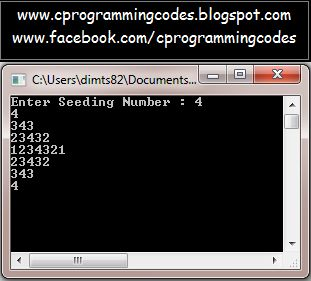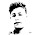## 1.31.2016

### Number Pattern of Half Diamond

Q. Write a C program to make number pattern of half diamond as:

4
343
23432
1234321
23432
343
4

Ans.

Before the writing the source code of above program, lookout the below figure:

4
343
23432
1234321
23432
343
4

You can see, there are four type pattern available in above figure.

If you are newbie in making the pyramid pattern programs in C, then you should firstly make the above 4 pyramid separately and after successfully making above 4 pyramid pattern, just merge them and do the merging action (i.e. some minor changes required when you merge pyramid). You are done. Cheers !!

Let's start to write the code for above number pattern of half diamond.

/*c program for number pattern of half diamond*/
#include<stdio.h>
int main()
{
int num,n,r,c,p,q;
printf("Enter Seeding Number : ");
scanf("%d", &num);
for(r=1,n=num; r<=num; r++,n--)
{
for(c=r,p=n; c>=1; c--,p++)
printf("%d",p);
for(c=r,p=num-1; c>1; c--,p--)
printf("%d",p);
printf("\n");
}
for(r=1; r<num; r++)
{
for(c=r,p=r+1; c<num; c++,p++)
printf("%d",p);
for(c=num-r,p=num-1; c>1; c--,p--)
printf("%d",p);
printf("\n");
}
getch();
return 0;
}

/*****************************************************
The output of above program would be:
******************************************************/Figure: Scree-shot for Number Pattern of Half Diamond C Program

Related Programs:
1. Character pattern of Half Diamond C program:
D
CDC
BCDCB
ABCDCBA
BCDCB
CDC
D

You might also like to read:

#### 1 comment:

1.There is a difference between &array and &array.
In point 2 it is mentioned that &array is an alias for &array.

But &array indicates the address of the entire array where as &array indicates the address of the first element in the array.

for example

int *p = &array; //gives a compiler error.

where as

int *ptr = &array; // is perfectly legal.